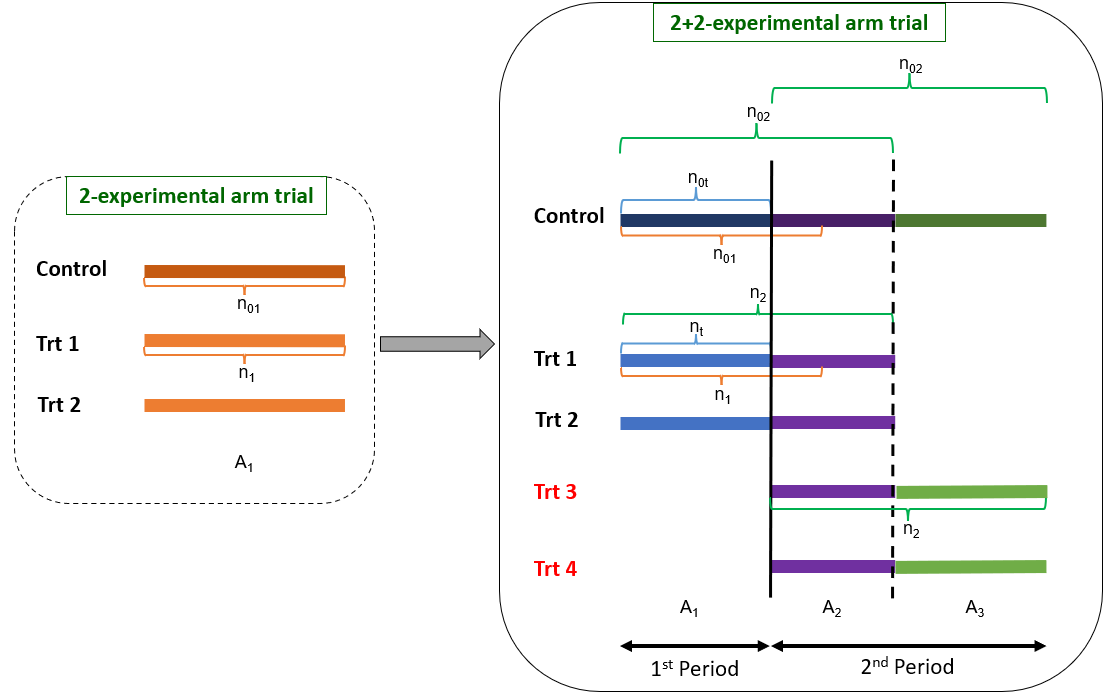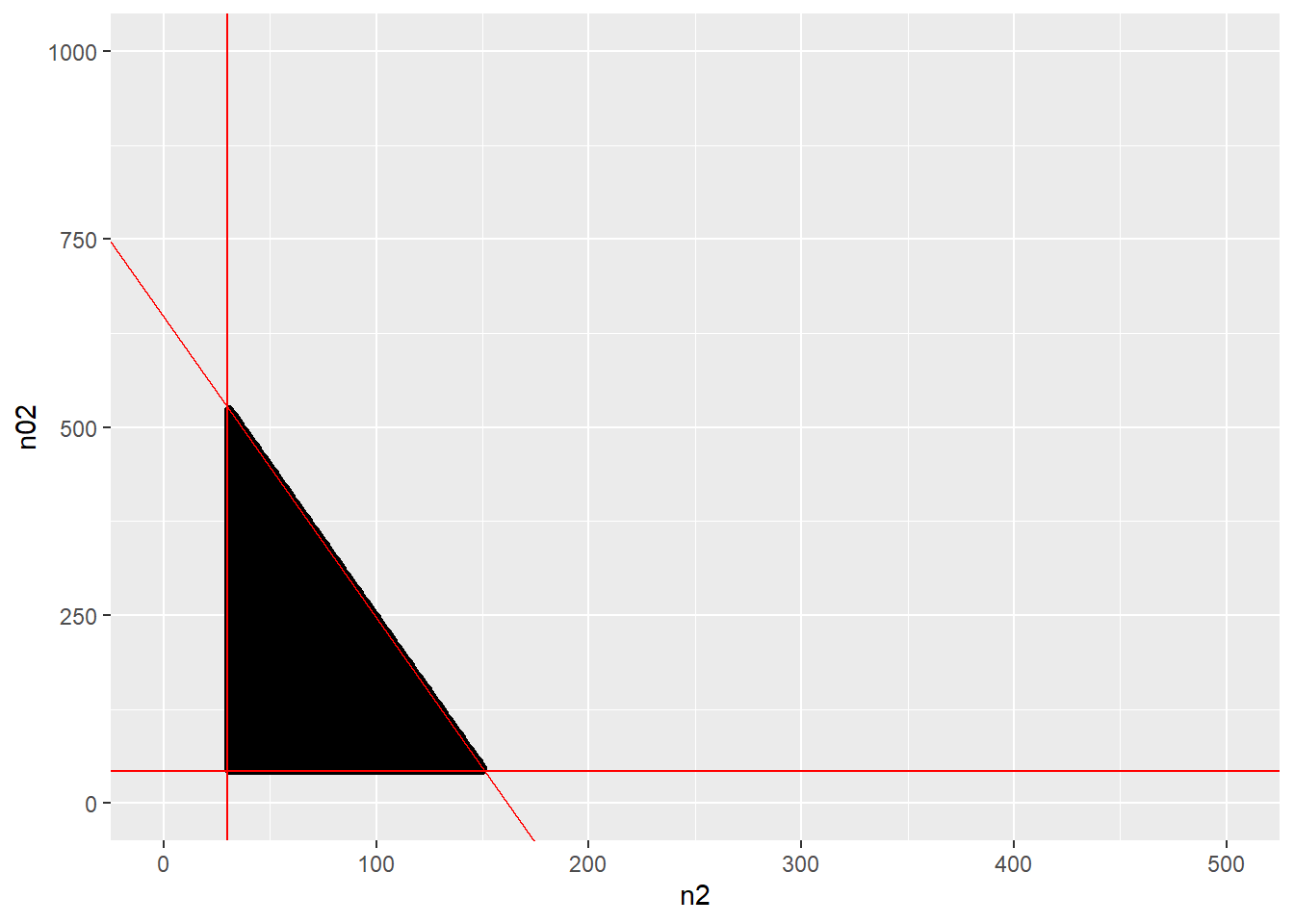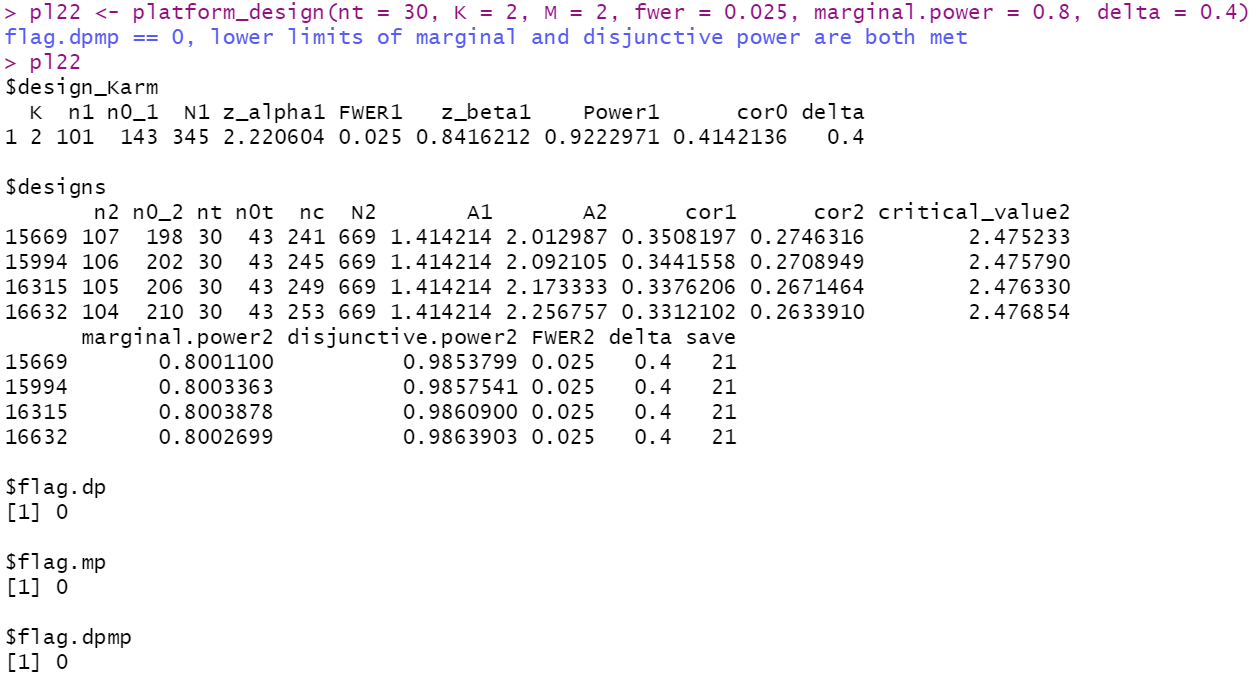library(PlatformDesign)
library(ggplot2)

This vignette provides a guidance for designing an optimal two-period K+M-experimental arm platform trial with M delayed experimental arms added during the trial using the package PlatformDesign, controlling for family-wise type-I error rate (FWER) or pair-wise type-I error rate (PWER). The K+M-experimental arm trial has K experimental arms and one control arm during the first period, and later M experimental arms are added on the start of the second period. The one common control arm is shared among all experimental arms across the trial. The design method suits any K+M-experimental arm trial, with the examples here shows how to design a 2+2-experimental arm trial (see Fig 1 for the scheme). The goal of the proposed method is to find the optimal design with a minimum total sample size while making the marginal and disjunctive power no less than their counterparts in the K-experimental arm trial it is based on, controlling for FWER or PWER.Fig 1.Schema of a two-period 2+2-experimental arm platform trial. Left part of the figure shows a traditional three-arm trial, in the context of this paper, we refer it as a 2-experimental arm trial as it has 2 experimental arms and 1 common control. In the right part of this figure, it is the 2+2-experimental arm trial. During the first period, the 2+2-experimental arm trial has two experimental arms, Trt 1 and Trt 2 (light blue segments), and a control arm (the dark blue segment). The first vertical line (solid) separates the first and second period of the 2+2-experimental arm trial, and indicates the opening of the two new experimental arms, Trt 3 and Trt 4. The second vertical line (dashed) separates two parts of the second period, and indicates the closing of Trt 1 and Trt 2. In the first part of the second period, the control arm (the dark purple segment) is shared among the four experimental arms (light purple segments). During the second part of the second period, the control (dark green), Trt 3, and Trt 4 (light green) continue to accrue patients until reaching the planned sample sizes. The nt and n0t (blue brackets) indicate the numbers of patients enrolled in each of Trt 1 and Trt 2, and the control, respectively, when Trt 3 and Trt 4 are added. The n1 and n01 (orange brackets) indicate the sample sizes for each of the two experimental arms and the control, respectively, in the 2-experimental arm trial. The n2 and n02 (green brackets) indicate the sample sizes for each of the four experimental arms and their corresponding concurrent control in the two-period 2+2-experimental arm trial. A1 denotes the allocation ratio (control to experimental arm) during the first period of the 2+2-experimental arm trial. A2 denotes the allocation ratio during the the first part of the second period, when all four experiments arms are open. A3 is equal to A1.

## Example 1: the optimal two-period multiarm trial design with delayed arms, controlling for FWER

The following steps contain two parts: 1) Steps 1 to 5 derive the design parameters in the K-experimental arm trial that the K+M-experimental arm trial is based on. The K+M-experimental arm trial is based on the K-experimental arm trial in the sense that we will keep the same FWER and marginal power for the K+M-experimental arm trial as in the K-experimental arm trial, despite M new experimental arms are added during the second period of the K+M experimental arm trial. 2) Steps 6 to 14 illustrate how the design parameters are calculated for the K+M-experimental arm trial.

For users who are less interested in the theoretical details, you can skip other steps and focus on Steps 1, 7, 13, and 14.

### Step 1: initial setup

Four design parameters for the K-experimental arm trial should be pre-specified: the number of experimental arms ($$K$$), the family-wise error rate ($$FWER_1$$), the marginal type II error ($$\beta_1$$), and the allocation ratio (control-to-each experimental arm, denoted as $$A_1$$). In our method, we use $$A_1=\sqrt{K}$$, according to the K-root optimal allocation rule. In the following code, we assume $$K=2$$, $$FWER_1=0.025$$, $$\beta_1=0.2$$, and $$A_1=\sqrt{2}$$. In addition, $$z_{\beta_1}$$ ( in the following code) is the corresponding critical value for the power of $$1-\beta_1$$.

K <- 2
FWER_1 <- 0.025
beta1 <- 0.2
z_beta1 <- qnorm(1-beta1) #z_(1-beta1)
A1 <- sqrt(K)

### Step 2: Correlation Matrix 1

We use $$Z_1$$ and $$Z_2$$ to denote the two test statistics for comparing the two experimental arm to the control in the original design. Given $$A_1$$, the correlation between $$Z_1$$ and $$Z_2$$ (denoted as $$\rho_0$$) and the correlation matrix (denoted as $$\Sigma_{1}$$) can be calculated (see below).

First, we have, $\rho_{0} = \frac{n_{0kk^{'}}}{\frac{(n_{01})^{2}}{n_{1}}+n_{01}}$ where $$n_1$$ is the number of patients on each of the experimental arm, $$n_{01}$$ is the number of patients on the control in the K-experimental arm trial, and $$n_{0kk^{\prime}}$$ is number of control patients shared between 2 experimental arms $$k$$ and $$k'$$.

Since the two experimental arms share a common control arm, that is, $$n_{0kk^{'}}=n_{01}$$. The correlation of $$Z_1$$ and $$Z_2$$ is computed as $\rho_0 = \frac{1}{(n_{01}/n_{1}+1)}=\frac{1}{(A_1+1)}=0.4142$

We can see that in our “2+2”-experimental arm example, where K=2, we have $\Sigma_{1} =\begin{bmatrix} 1& \rho_0\\ \rho_0& 1 \end{bmatrix}=\begin{bmatrix} 1& 0.4142\\ 0.4142& 1 \end{bmatrix}$

Based on the above description, the function one_stage_multiarm can be used to find $$\rho_0$$ and the correlation matrix $$\Sigma_1$$ of $$Z_1$$ and $$Z_2$$ as shown below.

multi <- one_stage_multiarm(K = 2, fwer = 0.025, marginal.power = 0.8, delta = 0.4)
corMat1 <- multi$corMat1 corMat1 #> [,1] [,2] #> [1,] 1.0000000 0.4142136 #> [2,] 0.4142136 1.0000000 ### Step 3: Critical Value 1 Given $$K$$, $$\Sigma_{1}$$ and $$FWER_1$$, we can find the associated critical value (denoted as $$z_{1-\alpha_1}$$) for the marginal type I error rate in the 2-experimental arm trial (denoted as $$\alpha_1$$) based on the following equation. $FWER_1=1-\int_{-\infty }^{z_{1-\alpha_1}}\int_{-\infty }^{z_{1-\alpha_1}}...\int_{-\infty }^{z_{1-\alpha_1}}\pi _{z}(Z(z_{1},z_{2},...z_{K}), 0, \Sigma_1 )dz_{1}dz_{2}...dz_{K}$ This calculation can also be achieved using the function one_stage_multiarm. multi$z_alpha1
#>  2.220604

### Step 4: Sample Sizes 1

Given $$\beta_1$$, $$A_1=\sqrt{K}$$, an effective standardized effect size $$\Delta$$ (assumed to be 0.4 for all experimental arms in our paper), and $$z_{1-\alpha_1}$$ derived above, we can derive sample sizes for the experimental ($$n_1$$) and control arms ($$n_{0_1}=A_1n_1=\sqrt{K}n_1$$), respectively, as shown below.

Since we have

$z_{1-\alpha_1}+z_{1-\beta_1}=\frac{\mu_i-\mu_0}{\sigma\sqrt{\frac{1}{n_{1}}+\frac{1}{\sqrt{K}n_{1}}}}=\frac{\Delta }{\sqrt{\frac{1}{n_{1}}+\frac{1}{\sqrt{K}n_{1}}}}$ Therefore,

$n_{1}= \frac{(z_{\alpha_1}+z_{\beta_1})^2}{\Delta^2}(1+\frac{\sqrt{K})}{K})$

and $n_{0_{1}}= \frac{(z_{\alpha_1}+z_{\beta_1})^2}{\Delta^2}(1+\sqrt{K})$

In sum, the total sample size of the K-experimental arm trial is $N_1=Kn_{1}+n_{0_{1}}$

With our “2+2” example, we again use the function one_stage_multiarm to derive the sample sizes for the first period.

multi$n1 #>  101 multi$n0_1
#>  143
multi$N1 #>  345 ### Step 5: Disjunctive Power 1 With $$\beta_1$$ and $$\Sigma_{1}$$, we can also derive the overall (disjunctive) power $$\Omega1=0.922$$ based on equation below. $\Omega_{1}=1-\int_{-\infty }^{z_{\beta1}}\int_{-\infty }^{z_{\beta1}}...\int_{-\infty }^{z_{\beta1}}\pi _{z}(Z(z_{1},z_{2},...z_{K}), 0, \sum )dz_{1}dz_{2}...dz_{K}$ This result is also included as part of the output from the function one_stage_multiarm, i.e., $Power1.

multi$Power1 #>  0.9222971 From the above outputs, we can see that the computed disjunctive power is 0.922. Based Steps 1 to 5, we demonstrated given $$K, FWER_1$$, marginal power $$1-\beta_1$$ and the standardized effect size $$\Delta$$, how to derive the marginal type I error rate $$\alpha_1$$ and its corresponding critical value, sample size $$n_1$$ for each of the experimental arm and $$n_{0_1}$$ for the control arm, and disjunctive power $$\Omega_1$$ for the K-experimental arm trial. In sum, the function one_stage_multiarm in R package PlatformDesign can complete step 1 to 5 all at once. Below are the complete outputs generated by applying this function. multi #>$K
#>  2
#>
#> $n1 #>  101 #> #>$n0_1
#>  143
#>
#> $N1 #>  345 #> #>$z_alpha1
#>  2.220604
#>
#> $FWER1 #>  0.025 #> #>$z_beta1
#>  0.8416212
#>
#> $Power1 #>  0.9222971 #> #>$corMat1
#>           [,1]      [,2]
#> [1,] 1.0000000 0.4142136
#> [2,] 0.4142136 1.0000000
#>
#> $delta #>  0.4 Based on these knowledge, we can introduce our proposed methods when adding new arms in the following from steps 6 to 14. ### Step 6: Timing of Adding New Arms Timing is the first component to consider if planning to add new experimental arms during a study. In practice, we can use a fraction to denote the timing. In this paper, we use the number of patients already being enrolled in the experimental arm (denoted as $$n_t$$) when new arms added to define the timing of adding. By this definition, at the time of adding new arms, number of patients enrolled in the control arm is $$n_{0t}=[A_1n_t]$$. For example, the following codes describe a scenario if new arms are added when there have 30 patients enrolled for each of the experimental arms. nt <- 30 nt #>  30 n_0t <- ceiling(nt*A1) n_0t #>  43 ### Step 7: Initial Setup 2 Then we need to decide the family-wise error rate when adding new arms, denoted as $$FWER_2$$. In this example, we control the $$FWER_2$$ at the same level to the $$FWER_1$$. With $$FWER_2$$, we can find the marginal type I error rate (denoted as $$\alpha_1$$). With $$\alpha_1$$, we can find the updated marginal power (denoted as $$\omega_2=1-\beta_2$$), and then the disjunctive power (denoted as $$\Omega_2$$). We will describe these updates with details in the following steps. The goal of a two-period K+M experimental arm platform design is to minimize the sample size (denoted as $$N_2$$) while keeping the marginal power ($$\omega_2$$) and disjunctive power ($$\Omega_2$$) no less than their counterparts in the first period ($$\omega_1$$ and $$\Omega_1$$). That is, we set the lower limit of $$\omega_2$$ (denoted as $$\omega_{2min}$$) to be 0.8, the lower limit of $$\Omega_2$$ (denoted as $$\Omega_{2min}$$) to be 0.922 (which is obtained by using function one_stage_multiarm in the previous steps) as for our “2+2” example. These two limits will be used to select the recommended optimal design(s) (details shown in Step 13). FWER_2 <- FWER_1 FWER_2 #>  0.025 omega2_min <- 1-beta1 omega2_min #>  0.8 Omega2_min <- multi$Power1
Omega2_min
#>  0.9222971

Because we need to keep $$FWER_2$$ equal to $$FWER_1$$ when adding new arms, we then have to update $$n_1$$ to $$n_2$$ and $$n_{01}$$ to $$n_{02}$$ for each experimental arm (See Fig 1). Here $$n_{2}$$ and $$n_{02}$$ are the sample sizes for each of the experimental arms and for the concurrent control in the 2+2-experimental arm trial.

We define an admissible set for pairs of $$(n_{2}, n_{02})$$ based on the following three constraints. The first two constraints for $$(n_{2}, n_{02})$$ is related to the allocation ratio after adding the new arms, denoted as $$A_2$$. In the first period with two experimental arms (before adding the new arms), the control allocation ratio is $$A_1$$. Once the two new experimental arms are added, we need to use an allocation ratio $$A_2$$ to achieve desired design properties (e.g., control the FWER and achieve the marginal power). After the initially opened two experimental arms are completed, the trial will again have only two experimental arms left. Therefore, the allocation ratio will go back from $$A_2$$ to $$A_1$$.

Here we have,

$A_2=(n_{0_2}-n_{0t})/(n_2-n_t) > 0$

where $$n_t$$ and $$n_{0t}$$ are number of enrolled patients for each of the experimental arms and the control at the time of adding new arms. That is, the value of $$A_2$$ needs to be a non-infinite positive number. For example, the first two constraints in our “2+2” example are

$n_{0_2} > n_{0t}=43$ and $n_2>n_t=30$

We also need to set an upper limit for the total sample size $$N_2$$. A reasonable upper limit is that $$N_2$$ should not exceed the required sample sizes (denoted as $$S$$) of separately conducting two multiarm trials, i.e., a K-experimental arm trial and a M-experimental arm trial. To recap, $$K$$ and $$M$$ refer to the numbers of initially and newly added experimental arms.

Therefore, S can be calculated as $S= \frac{(z_{1-\alpha_1}+z_{1-\beta_1})^2}{\Delta^2}(1+2\sqrt{K}+K) + \frac{(z_{1-\alpha_1^*}+z_{1-\beta_1})^2}{\Delta^2}(1+2\sqrt{M}+M).$

, where $$z_{1-\alpha_1}$$ and $$z_{1-\alpha_1^*}$$ are the critical values for the K- and M-experimental arm trial, separately. In our “2+2” example, $$z_{1-\alpha_1}=z_{1-\alpha_1^*}$$ as $$K=M=2$$ and $$S=2N_1=690$$.

Therefore, the third constraint for $$(n_{2}, n_{0_{2}})$$ is $N_2=(K+M)n_2+n_{0_2}+n_{0t}<S=690.$

Under the above three constraints, the admissible set of $$(n_{2}, n_{0_{2}})$$ can be identified using the function admiss (integer points in triangle area in Fig.2). The data set pair3 contains all $$(n_{2}, n_{02})$$ pairs satisfying the 3 constraints introduced above.

pair3 <- admiss(n1=101, n0_1=143, nt=30, ntrt=4, S=690)

ggplot(data=pair3, aes(x=Var1, y=Var2)) +
geom_point() +
geom_abline(intercept = 647, slope=-4, color="red") +
geom_hline(yintercept=43, color="red")+
geom_vline(xintercept=30, color="red") +
xlim(0, 500)+
ylim(0,1000)+
xlab("n2")+
ylab("n02")### Step 9: Correlation Matrix 2

For each pair of $$(n_{2}, n_{0_{2}})$$ in the admissible set the correlation matrix of z statistics, $$\sum_{2}$$, of four test statistics $$(Z_1, Z_2, Z_3, and Z_4)$$ can be derived using in $$n_{2}$$ and $$n_{0_{2}}$$. The correlation between two experimental arms is,

$\rho_{k, k^{'}} = \frac{n_{0kk^{'}}}{\frac{(n_{0_{2}})^{2}}{n_{2}}+n_{0_{2}}}$

Specifically, between the initially opened two experimental arms (and between the two newly added arms), the shared control now is $$n_{0kk^{'}}=n_{02}$$. Therefore, the correlation of Z statistics between these two initially opened experimental arms (and between the two newly added arms) is

$\rho_1 = \frac{1}{(n_{0_2}/n_2+1)}$.

The number of shared controls between one initially opened experimental arm and one delayed experimental arm is $$n_{0kk^{'}}=n_{0_2}-n_{0t}$$ Therefore, the correlation of the Z test statistics between these two experimental arms is

$\rho_2 = \frac{n_{0_2}-n_{0t}}{(n_{0_2}^2/n_2+n_{0_2})}$.

To be specific, in our 2+2-experimental arm example, we have $$\Sigma_2$$ as, $\Sigma_2 =\begin{bmatrix} 1 & \rho_1 & \rho_2 & \rho_2\\ \rho_1 & 1& \rho_2& \rho_2\\ \rho_2 & \rho_2& 1& \rho_1\\ \rho_2 & \rho_2& \rho_1 & 1 \end{bmatrix}$

### Step 10: Critical Value 2

Now we can use $$FWER_2$$ and $$\Sigma_{2}$$ to calculate the marginal type I error $$\alpha_2$$ and the corresponding critical value $$z_{1-\alpha_2}$$ for each pair of $$(n_{2}, n_{02})$$ in the admissible set found in ).

$FWER_2=1-\int_{-\infty }^{z_{1-\alpha_2}}\int_{-\infty }^{z_{1-\alpha_2}}...\int_{-\infty }^{z_{1-\alpha_2}}\pi _{z}(Z(z_{1},z_{2},...z_{K+M}), 0, \Sigma_2 )dz_{1}dz_{2}...dz_{K+M}$

### Step 11: Marginal Power 2

With $$n_1$$, $$n_{01}$$, $${\alpha_1}$$, $${\beta_1}$$ and $${\alpha_2}$$, we can use the following equation to calculate the marginal power $$\omega_2=1-\beta_2$$ for each pair of $$(n_{2}, n_{02})$$ from $$z_{1-\beta_2}$$.

$z_{1-\beta2 }=\sqrt{\frac{\frac{1} {n_1}+\frac{1}{n_{0_1}}}{\frac{1}{n_2}+\frac{1}{n_{0_2}}}}(z_{1-\alpha1} + z_{1-\beta1})- z_{1-\alpha2}$

### Step 12: Disjunctive Power 2

With $$\beta_2$$ and $$\Sigma_2$$, we can derive the new disjunctive power $$\Omega_2$$ for each pair of $$(n_{2}, n_{0_{2}})$$ using the following equation.

$\Omega_{2}=1-\int_{-\infty }^{z_{\beta2}}\int_{-\infty }^{z_{\beta2}}...\int_{-\infty }^{z_{\beta2}}\pi _{z}(Z(z_{1},z_{2},...z_{K+M}), 0, \Sigma_2 )dz_{1}dz_{2}...dz_{K+M}$

### Step 13: Design Selection

In our “2+2” example, we calculate $$\omega_2$$ and $$\Omega_2$$ from all of the 29040 pairs of $$(n_2,n_{02})$$ in the entire admissible set. We then perform a 2-step selection procedure to obtain the recommended optimal design(s): 1) first we only keep the designs with $$\omega_2$$ and $$\Omega_2$$ above or equal to $$\omega_1$$ and $$\Omega_1$$, respectively (i.e., lower limits decided in Step 7); 2) next, among those selected ones we choose the designs with the smallest total sample size $$N_2$$.

Given $$n_t$$, $$K$$, $$M$$, $$FWER_1$$, $$\omega_1$$, and $$\Delta$$, the function platform_Design can provide the optimal designs with the minimum total sample size among those having $$\omega_2$$ and $$\Omega_2$$ no less than their counterparts in the K-experimental arm trial.

design <- platform_design(nt = 30, K = 2, M = 2, fwer = 0.025, marginal.power = 0.8, delta = 0.4)
design$designsFig 2. Recommended optimal designs when nt=30 in a 2+2-trial The first part of the outputs ($design Karm) contains the parameters for the K-experimental arm trial. The second part ($designs) contains the parameters for the K+M experimental arm trial designed based on the former. From above ($designs), we can see it is possible to have multiple recommended designs which all have the same total sample size N2. We provide a full list of useful parameters for each of the recommended optimal designs.

For example, if we choose design # 15669 for this 2+2-experimental arm trial, the corresponding critical value for controlling the $$FWER$$ at 0.025 is 2.475. The marginal power is 0.8, and the disjunctive power is 0.985, both no less than their counterparts in the 2-experimental arm trial. The required total sample size is $$N_2 = 669$$. Among the 669 patients, in the first period the sample sizes for each experimental arm and the control are $$n_t = 30$$ and $$n_{0t} = 43$$, with an allocation ratio of $$A_1 = 1.414$$. Once the two additional experimental arms are added, the optimal allocation ratio changes to $$A2 = 2.01$$ for the overlapping stage of the second period. The allocation ratio will change back to $$A_1$$ once the two initial experimental arms closed to accrual. Through the entire 2+2-experimental arm trial, the sample size for each experimental arm is $$n_2 = 107$$. The sample size for the concurrent control of each experimental arm is $$n_{02} = 198$$. To be noted, the sample size for the entire control arm, concurrent and non-concurrent combined, is $$n_c$$ = 241. The reduction in the total sample size comparing to two separate 2-experimental arm trials is 21.

### Step 14: Final Decision

As we can see from Step 13, although the total sample size is the same for all the 4 recommended designs, the other parameters can be different. Therefore, we can choose a final design based on our needs according to the other parameters. For example, if we would like to choose a design with the largest disjunctive power $$\Omega_2$$, our final choice goes to the design in the last row of the result above (design #16632).